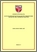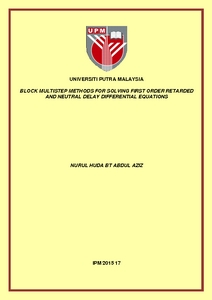# Block multistep methods for solving first order retarded and neutral delay differential equations

## Citation

Abdul Aziz, Nurul Huda (2015) Block multistep methods for solving first order retarded and neutral delay differential equations. PhD thesis, Universiti Putra Malaysia.

## Abstract

This thesis investigates the numerical solutions for solving first order retarded and neutral delay differential equations and its analysis in block multistep methods. The investigation begins by solving the retarded delay differential equations (RDDE) using 1-point multistep method and it then extended to the 2-point and 3-point block multistep methods. There are two strategies that have been implemented in the numerical solutions which are the variable step size and the variable step size and variable order. In the developed algorithm, a Newton divided difference interpolation has been used in approximating a non-vanishing delay, whilst a new approach that is based on an adaptation of predictor-corrector scheme has been used to handle the vanishing delay problems. Special attention according to the numerical treatment of discontinuity in the delay solution is also presented where the techniques developed is from the adaptation of ‘detect, locate and treat’ strategy. The results of these strategies are shown that it can be applied in the block multistep methods and able to solve even a type of state-dependent delays. The numerical solution of RDDE is then extended for solving the neutral delay differential equations (NDDE) of non-discontinuity and discontinuity cases. A new subroutine function has been added in the developed algorithm with the capabilities to approximate the neutral delay term using the interpolation of function evaluation. The interpolation accuracy that has been obtained from this approach is reliable and has an advantage in the less computational work. The analysis of all numerical methods including order, error constant, consistency,zero-stability and convergence properties are also have been presented. The proposed methods have shown to have a convergence when the numerical solution approaches to the exact solution as the step size h tends to zero. From the stability properties, it has been determined that the block multistep methods of RDDE have P-stability and Q-stability regions, whilst for the NDDE has NP-stability regions as the regions shrink when the step size ratios decreased. In conclusion, the performance of the proposed methods and the developed approaches are reliable and suitable for solving both retarded and neutral delay differential equations. Some advantages in terms of total number of steps and function calls of these proposed methods also have been identified when the comparison of the numerical results are made with the existing methods.

## Download FilePreview
PDF
IPM 2015 17IR.pdf

Download (944kB) | Preview

## Additional Metadata

Item Type: Thesis (PhD) Differential equations - Numerical solutions Numerical analysis IPM 2015 17 Zanariah Abdul Majid, PhD Institute for Mathematical Research Haridan Mohd Jais 14 Feb 2018 01:35 14 Feb 2018 01:35 http://psasir.upm.edu.my/id/eprint/58931 View Download Statistic

### Actions (login required)View Item